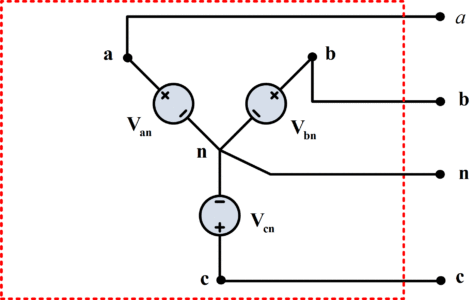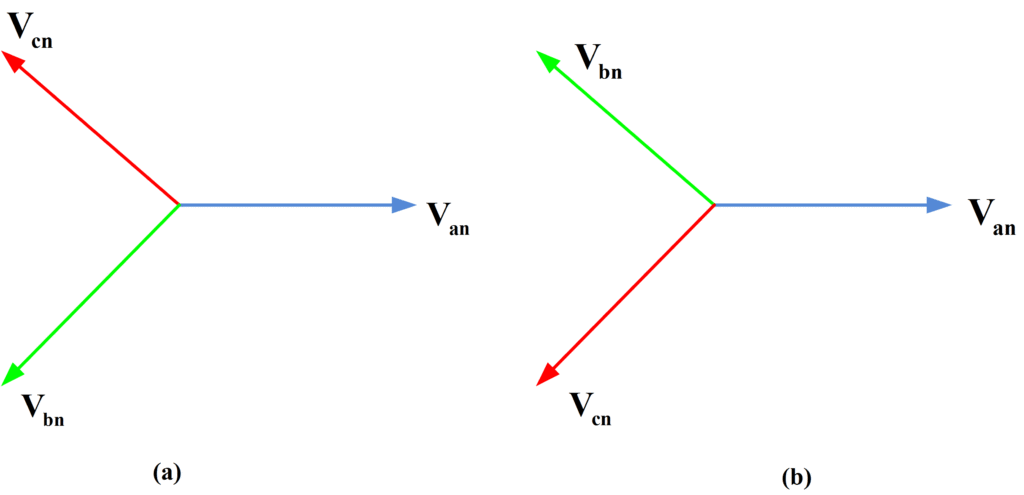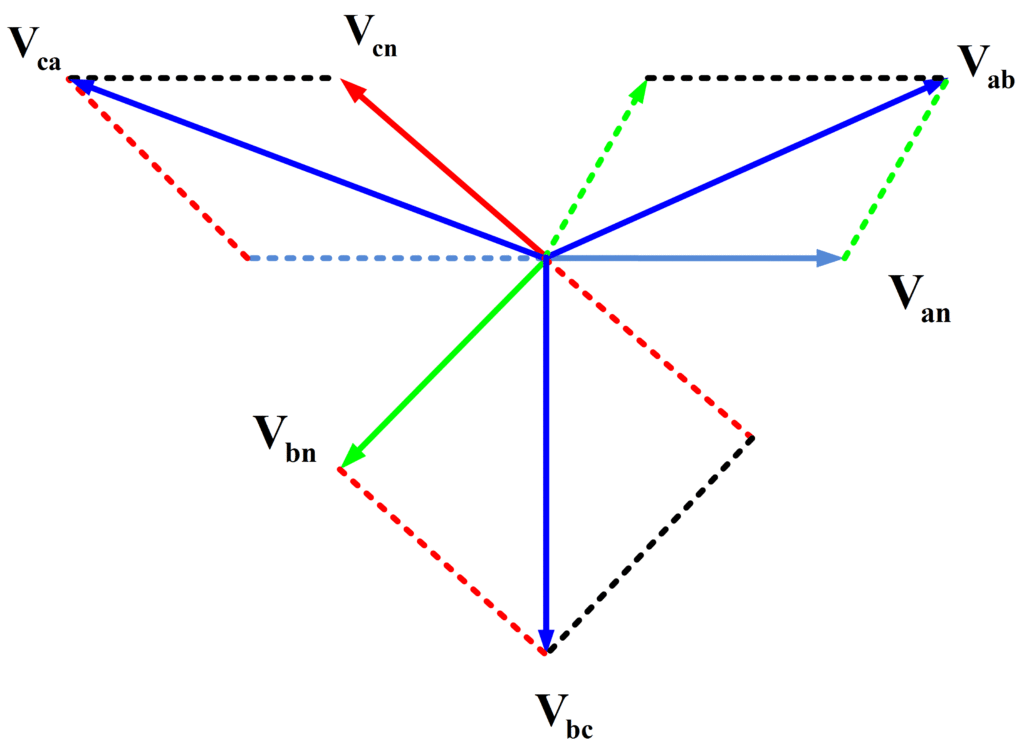# Three Phase Star Connection (Y): Three Phase Power,Voltage,Current

Want create site? Find Free WordPress Themes and plugins.

## Star Connection

In star connections, fundamentally we connect the same phase sides to a mutual (common) point known as neutral point and provide supply to its free ends which stay thereafter as shown in figure 1. As far as line and phase voltages are concerned, they are related to each other as:

${{\text{V}}_{\text{line}}}\text{=}\sqrt{\text{3}}{{\text{V}}_{\text{phase}}}$

Which means that whatever supply voltage we have, we need to insulate the windings for ${}^{1}/{}_{\sqrt{3}}$ times the line voltage only. Whereas, in a Star connection, line and phase currents remain the same as:

${{\text{I}}_{\text{line}}}\text{=}{{\text{I}}_{\text{phase}}}$

Let us consider the three-phase source of figure 1, which has line terminals a, b, and c and a neutral terminal n. in this case, the source is said to be Y-connected.Fig.1: Y-Connected Source

## Star Connection Line and Phase Voltages

The voltages Van, Vbn, and Vcn between the line terminals and the neutral terminal are called phase voltages and in most cases, we shall consider are given by

Phase Voltages for Star (Wye) Connection\begin{matrix} \begin{align} & {{V}_{an}}={{V}_{p}}\angle {{0}^{o}} \\ & {{V}_{bn}}={{V}_{p}}\angle -{{120}^{o}} \\ & {{V}_{cn}}={{V}_{p}}\angle {{120}^{o}} \\\end{align} & \cdots & (1) \\\end{matrix}

Or

\begin{matrix} \begin{align} & {{V}_{an}}={{V}_{p}}\angle {{0}^{o}} \\ & {{V}_{bn}}={{V}_{p}}\angle {{120}^{o}} \\ & {{V}_{cn}}={{V}_{p}}\angle -{{120}^{o}} \\\end{align} & \cdots & (2) \\\end{matrix}

In both cases, each phase voltage has the same RMS magnitude Vp, and the phases are displaced 120o, with Van arbitrarily selected as the reference phasor. Such a set of voltages is called a balanced set and is characterized by

$\begin{matrix} {{V}_{an}}+{{V}_{bn}}+{{V}_{cn}}=0 & \cdots & (3) \\\end{matrix}$

As may be seen from (1) or (2).

The sequence of voltages in (1) is called the positive sequence, or ABC sequence, while that of (2) is called the negative, or ACB sequence. Phasor diagrams of two sequences are shown in figure 2, where we may see by inspection that (3) holds. Evidently, the only difference between the positive and the negative sequence is the arbitrary choice of terminal labels a,b, and c. this without loss of generality i shall consider only the positive sequence.Fig.2: (a) Positive and (b) Negative Sequence

By (1), the voltages in ABC sequence may each be related to Van. The relationships are

\begin{matrix} \begin{align} & {{V}_{bn}}={{V}_{an}}\angle -{{120}^{o}} \\ & {{V}_{cn}}={{V}_{an}}\angle {{120}^{o}} \\\end{align} & \cdots & (4) \\\end{matrix}

The line-to-line voltages, or simply line voltages, in figure (1) are Vab, Vbc, and Vca, which may be found from the phase voltages. For example

\begin{align} & {{V}_{ab}}={{V}_{an}}+{{V}_{nb}} \\ & ={{V}_{p}}\angle {{0}^{o}}-{{V}_{p}}\angle -{{120}^{o}} \\ & =\sqrt{3}{{V}_{p}}\angle {{30}^{o}} \\\end{align}

In line manner,

${{V}_{bc}}=\sqrt{3}{{V}_{p}}\angle -{{90}^{o}}$

${{V}_{ca}}=\sqrt{3}{{V}_{p}}\angle -{{210}^{o}}$

If we denote the magnitude of the line voltages by VL, then we have

$\begin{matrix} {{V}_{L}}=\sqrt{3}{{V}_{p}} & \cdots & (5) \\\end{matrix}$

And thus

Line Voltages for Star (Wye) Connection\begin{matrix} \begin{align} & {{V}_{ab}}={{V}_{L}}\angle {{30}^{o}} \\ & {{V}_{bc}}={{V}_{L}}\angle -{{90}^{o}} \\ & {{V}_{ca}}={{V}_{L}}\angle -{{210}^{o}} \\\end{align} & \cdots & (6) \\\end{matrix}

These results may also be obtained graphically from the phasor diagram shown in figure (3).Fig.3: Phasor Diagram showing phase and line voltages

## Star Connection Line and Phase Currents

Let us consider the system of the figure (4), which is balanced Y-Y, three-phase, four wire system if the source voltages are given by (1). The term Y-Y applies since both the source and the load are Y-connected. The system is said to be balanced since the source voltages constitute a balanced set and the load is balanced (each phase impedance Zp is equal). The fourth wire is the neutral line n-N, which may be omitted to form a Three-phase, three-wire system.Fig. 4: Balanced Y-Y System

The line currents of figure 4 are evidently

\begin{matrix} \begin{align} & {{I}_{aA}}=\frac{{{V}_{an}}}{{{Z}_{p}}} \\ & {{I}_{bB}}=\frac{{{V}_{bn}}}{{{Z}_{p}}}=\frac{{{V}_{an}}\angle -{{120}^{o}}}{{{Z}_{p}}}={{I}_{aA}}\angle -{{120}^{o}} \\ & {{I}_{cC}}=\frac{{{V}_{cn}}}{{{Z}_{p}}}=\frac{{{V}_{an}}\angle {{120}^{o}}}{{{Z}_{p}}}={{I}_{aA}}\angle {{120}^{o}} \\\end{align} & \cdots & (7) \\\end{matrix}

The last two results are a consequence of (4) and show that the line currents also form a balanced set. Therefore their sum is

$-{{I}_{nN}}={{I}_{aA}}+{{I}_{bB}}+{{I}_{cC}}=0$

Thus the neutral carries no current in a balanced Y-Y four-wire system.

In the case of Y-connect loads, the currents in the lines aA, bB, and cC are also the phase currents ( the currents carried by the phase impedances). If the magnitudes of the phase and line currents are Ip and IL, respectively, then IL=Ip, and (7) becomes

Line and Phase Currents in Star (Wye) Connection\begin{matrix} \begin{align} & {{I}_{aA}}={{I}_{L}}\angle -\theta ={{I}_{p}}\angle -\theta \\ & {{I}_{bB}}={{I}_{L}}\angle -\theta -{{120}^{o}}={{I}_{p}}\angle -\theta -{{120}^{o}} \\ & {{I}_{cC}}={{I}_{L}}\angle -\theta +{{120}^{o}}={{I}_{p}}\angle -\theta +{{120}^{o}} \\\end{align} & \cdots & (8) \\\end{matrix}

Where θ is the angle of Zp.

## Star Connection Three Phase Power

The average power Pp delivered to each phase of figure 4 is

\begin{matrix} \begin{align} & {{P}_{p}}={{V}_{p}}{{I}_{p}}\cos \theta \\ & =I_{p}^{2}\operatorname{Re}({{Z}_{p}}) \\\end{align} & \cdots & (9) \\\end{matrix}

And the total power delivered to the load is

${{P}_{p}}=3{{P}_{p}}$

The angle θ of the phase impedance is thus the power factor angle of the three-phase load as well as that of a single phase.

Did you find apk for android? You can find new Free Android Games and apps.